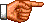## TUTORIAL ON MASTERMIND CODEBREAKING STRATEGY (1) (CODE MAY CONTAIN BLANK SPACES)

### Readers are advised to read "Tutorial on Mastermind Codebreaking Strategy (1)" before reading this webpage so that he / she may have a general understanding of the basic principles of Mastermind Codebreaking.

Game Setting: No. of colours: 7; Start from: Trial 1; Length of code: Unspecified (3 - 5); Hints contain error(s)?: No error; Order type: Fixed order; Colour repeated?: No repetition

Code: 246bb

As blank spaces (hereinafter represented by the letter b) are not considered as one type of colours and are not counted when giving marks, they must be specially treated. My strategy is to delay the use of blank spaces as much as possible, i.e. blank spaces are only used when there is no alternative. Let's look at an example for the application of this strategy.

In the beginning we can input any 5 digits. Suppose we input 01234 and the hint we get is 2. At the end of the 1st Round, we get the following Trace Table:

RoundInputHintNew Digits Used in this RoundDigit(s) Assumed to be Correct
101234201234-

Then we enter the 2nd Round and review our guess result in the 1st Round first. As we have got 2 digits correct in the 1st Round, we first assume that those 2 digits are 01. In addition, we need 3 new digits. But now there are only two digits for us to use (5 and 6) and under the present game setting, we can use at most two blank spaces. So we use one blank space to fill the remaining vacant positions, i.e. we input 0156b in the 2nd Round and the hint is 1. At the end of the 2nd Round, our Trace Table becomes:

RoundInputHintNew Digits Used in this RoundDigit(s) Assumed to be Correct
10123420123401
20156b156-

Then we enter the 3rd Round and review our guess result in the 2nd Round first. As in the 2nd Round we assumed that 01 was correct but now the hint was only 1, this shows that our previous assumption is incorrect. And so we return to the 1st Round and change our previous assumption to 02 and then proceed forward to the 2nd Round again. Comparing 02 and 0156b, we conclude that if the Code really contains 02, then we should have at least 1 correct digit in the 2nd Round, and the hint for the 2nd Round is exactly 1. In other words, 56 is not included in the Code. But then there is no new digit for us to use and we can only use two blank spaces at most, which is not enough to fill the vacant positions. This shows that our previous assumption is incorrect and so we return to the 1st Round again and modify our assumption. The readers should find that in case we change the assumption in the 1st Round to 03, 04, 12, 13 or 14, we cannot find enough digits or blank spaces to fill the remaining vacant positions (readers are advised to check this themselves). So next we try 23 and see if we can carry on our reasoning based on this assumption.

Comparing 23 and 0156b, we conclude that if the Code really contains 23, then there should be no correct digit in the 2nd Round. Since the hint for the 2nd Round is 1, which is one more than what we expected, we may assume that this digit is 5. As we now assume that the correct digits are 235 and there is no new digit for us to use, we may use two blank spaces to fill the remaining vacant positions. So we input 235bb and the hint is 1. At the end of the 3rd Round, our Trace Table becomes:

RoundInputHintNew Digits Used in this RoundDigit(s) Assumed to be Correct
10123420123423
20156b1565
3235bb1--

Next we enter the 4th Round and review the guess result in the 3rd Round first. As in the 3rd Round we assumed that 235 was correct but the hint was only 1, this shows that our previous assumptions are incorrect. So we return to the 2nd Round first and change the assumption to 6, and then proceed forward to the 3rd Round. Comparing 236 and 235bb, we conclude that if the Code really contains 236, then we should get at least 2 correct digits in the 3rd Round. But the hint for that Round is 1, which shows that our assumptions are still incorrect. We then return to the 1st Round and change the assumption to 24, and then proceed forward to the 2nd Round. Comparing 24 and 0156b, we conclude that if the Code really contains 24, then we should get no correct digit in the 2nd Round. But the hint for that Round is 1, which is one more than what we expected. We may assume that the digit is 5 and then proceed forward to the 3rd Round. Comparing 245 and 235bb, we conclude that if the Code really contains 245, then we should get at least 2 correct digits. But the hint for that Round is 1. This shows that our assumptions are still incorrect. So we return to the 2nd Round once again and change the assumption to 6. Comparing 246 and 235bb, we conclude that if the Code really contains 246, then we should get at least 1 correct digit in the 3rd Round. The hint for that Round is exactly 1, which is consistent with our assumptions. As we now assume that 246 are the correct digits, there are still two vacant positions, which may be filled by two blank spaces, i.e. we input 246bb as our guess. As a result, we break the Code. At the end of the 4th Round, our Trace Table becomes:

RoundInputHintNew Digits Used in this RoundDigit(s) Assumed to be Correct
10123420123424
20156b1566
3235bb1--
4246bbWIN--Link to Mastermind Link Page.